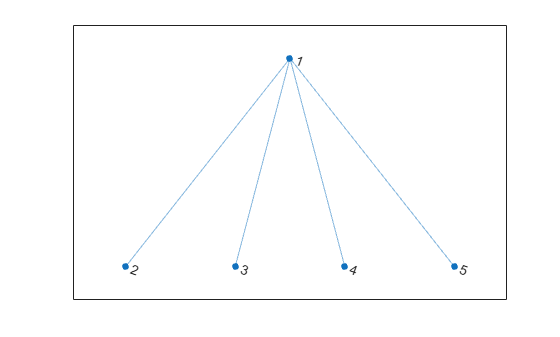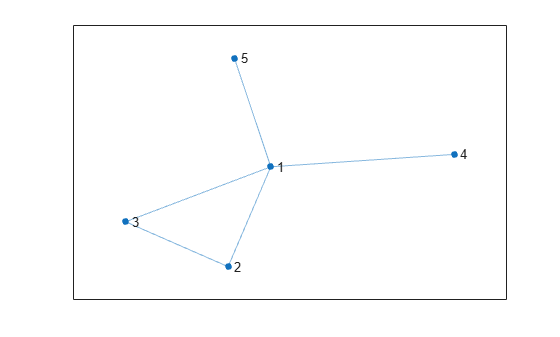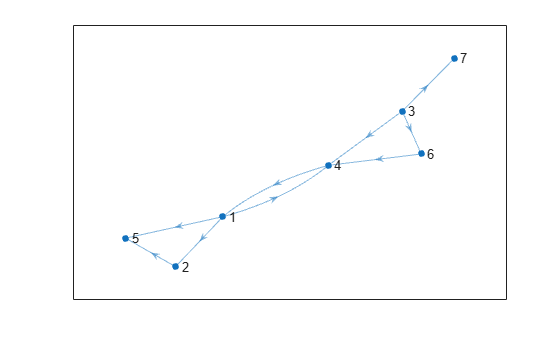# hascycles

Determine whether graph contains cycles

## Syntax

``tf = hascycles(G)``

## Description

example

````tf = hascycles(G)` returns logical `1` (`true`) if graph `G` contains one or more cycles, and logical `0` (`false`) otherwise.```

## Examples

collapse all

Create and plot an undirected graph.

```G = graph([1 1 1 1],[2 3 4 5]); plot(G)```Determine whether the graph has cycles.

`tf = hascycles(G)`
```tf = logical 0 ```

Now add an edge to the graph between node 2 and node 3. Replot the graph.

```G = addedge(G,2,3); plot(G)```Determine whether the new graph has cycles.

`tf2 = hascycles(G)`
```tf2 = logical 1 ```

Examine the difference between the `hascycles` and `isdag` functions operating on a directed graph.

Create and plot a directed graph.

```s = [1 1 1 2 3 3 3 4 6]; t = [2 4 5 5 6 7 4 1 4]; G = digraph(s,t); plot(G)```Determine whether the graph contains any cycles.

`tf = hascycles(G)`
```tf = logical 1 ```

`hascycles` returns `true` when a directed graph contains a cycle.

Now, use `isdag` to determine whether the graph is directed and acyclic.

`tf2 = isdag(G)`
```tf2 = logical 0 ```

`isdag` returns `false` because the graph contains a cycle. In general, the `hascycles` and `isdag` functions return opposite results for directed graphs.

## Input Arguments

collapse all

Input graph, specified as either a `graph` or `digraph` object. Use `graph` to create an undirected graph or `digraph` to create a directed graph.

Example: `G = graph(1,2)`

Example: `G = digraph([1 2],[2 3])`

collapse all

### Graph Cycles

A cycle exists in a graph when there is a nonempty path in which only the first and last nodes are repeated. An example of a cycle is: (Node1 - Node2 - Node3 - Node1).

A cycle cannot traverse the same edge twice. For example, the cycle (Node1 - Node2 - Node1) in an undirected graph only exists if there is more than one edge connecting Node1 and Node2. By this definition, self-loops count as cycles, though they cannot be part of any larger cycles.

## Version History

Introduced in R2021a